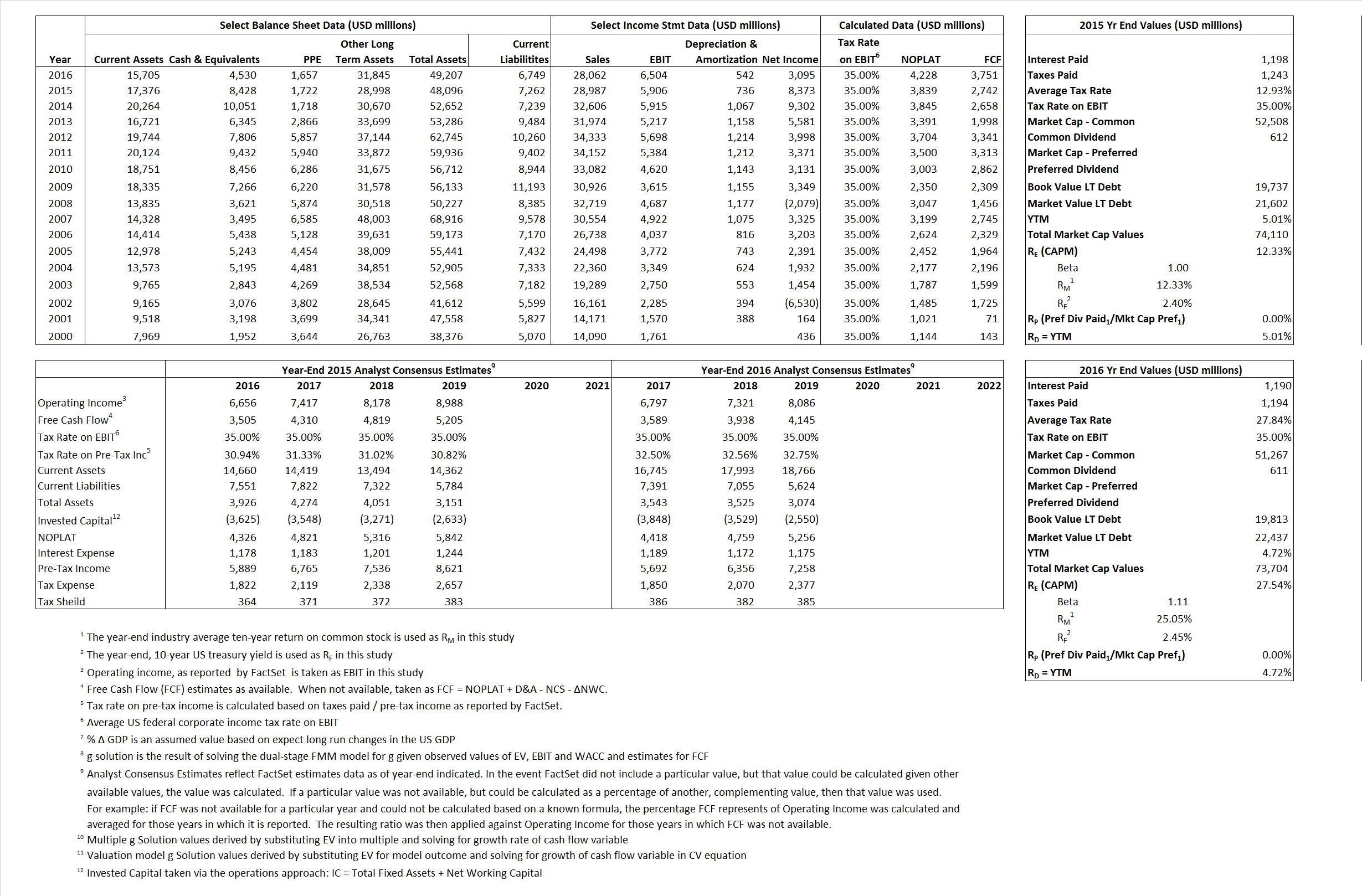# Twenty-First Century Fox Inc

## Analyst Listing

The following analysts provide coverage for the subject firm as of May 2016:

 Broker Analyst Analyst Email Pacific Crest Securities-KBCM Andy Hargreaves ahargreaves@pacific-crest.com Nomura Research Anthony DiClemente anthony.diclemente@nomura.com FBR Capital Markets & Co Barton Crockett bcrockett@fbr.com Stifel Nicolaus Benjamin E. Mogil bmogil@stifel.com Pivotal Research Group Brian Wieser brian@pvtl.com Deutsche Bank Research Bryan Kraft bryan.kraft@db.com BMO Capital Markets Daniel Salmon dan.salmon@bmo.com Topeka Capital Markets David W. Miller teagan@telseygroup.com Cowen & Company Doug Creutz doug.creutz@cowen.com Atlantic Equities Hamiton Faber h.faber@atlantic-equities.com Wedbush Securities James Dix james.dix@wedbush.com JBL Advisors Jeffrey B. Logsdon jeff@jbladvisors.com Jefferies John Janedis jjanedis@jefferies.com Needham Laura Martin lmartin@needhamco.com Wells Fargo Securities Marci Ryvicker marci.ryvicker@wellsfargo.com Guggenheim Securities Michael C. Morris michael.morris@guggenheimpartners.com Credit Suisse Omar Sheikh omar.sheikh@credit-suisse.com BTIG Richard Greenfield rgreenfield@btig.com RBC Capital Markets Steven Cahall steven.cahall@rbccm.com Bernstein Research Todd Juenger todd.juenger@bernstein.com Telsey Advisory Group Tom Eagan teagan@telseygroup.com Evercore ISI Vijay Jayant vijay.jayant@evercoreisi.com

## Primary Input Data## Derived Input Data

### Equational Form

Net Operating Profit Less Adjusted Taxes NOPLAT 3,839 4,228$NOPLAT\, =\, EBIT\, x\, (1 \,-\, Avg \,\,Tax\,\, Rate\,\, on\,\, EBIT)$
Free Cash Flow FCF 2,742 3,751$FCF\,=NOPLAT\,+\,Non-Cash\,Expenses-\Delta NWC\,-\,NCS$
Tax Shield TS 155 331$TS\,=\,Interest\,\,Paid\,\,x\,\, Avg \,\,Tax\,\,Rate\,\, on\,\, Pre-Tax\,\, Income$
Invested Capital IC 40,834 42,458$IC\,=\,Fixed\,\,Operating\,\,Assets\,\,+\,\,Net\,\, Working\,\, Capital$
Return on Invested Capital ROIC 9.40% 9.96%$ROIC\,=\,\frac { NOPLAT }{ IC }$
Net Investment NetInv (3,843) 2,166$NetInv\,=\,{ {IC}_{1}}-{{IC}_{0}}+Depreciation$
Investment Rate IR -100.11% 51.23%$IR\,=\,\frac {NetInv}{NOPLAT}$
Weighted Average Cost of Capital
WACCMarket 10.01%  20.19%$WACC\,=\,\frac { E }{ V } { R }_{ E }\,+\,\frac { P }{ V } { R }_{ P }\,+\,\frac { D }{ V } { R }_{ D }\left( 1- Avg\,\, Tax\,\,Rate\,\,on\,\,Pre-Tax\,\,Income \right)$
WACCBook   7.70%  7.51%
Enterprise value
EVMarket 65,682 69,174$EV\,=\,Market\,\,Cap\,\,Equity\,+\,\,Long\,\,Term\,\,Debt\,-\,Cash$
EVBook   63,817  66,550
Long-Run Growth
g = IR x ROIC
-9.41%  5.10% Long-run growth rates of the income variable are used in the Continuing Value portion of the valuation models.
g = %$\Delta$ GDP   2.50%   2.50%
Margin from Operations M   20.37%  23.18%$M\,\,=\,\,\frac{EBIT}{SALES}$
Depreciation/Amortization Rate D   11.08%  7.69%$D\,\,=\,\,\frac{D+A}{EBITDA}$

## Valuation Multiple Outcomes

The outcomes presented in this study are the result of original input data, derived data, and synthesized inputs.

### model g solution

12/31/2015 12/31/2016 12/31/2015 12/31/2016 12/31/2015 12/31/2016

EV/SALES$\frac {EV}{Sales} \,= \,\frac{ROIC\, -\, g}{ROIC\,(WACC\,-\,g)}\,(1\,-\,T)\,(M)$

2,27 2.47  11.00%  36.45%  10.72%  25.79%

EV/EBITDA$\frac {EV}{EBITDA} \,= \,\frac{ROIC\, -\, g}{ROIC\,(WACC\,-\,g)}\,(1\,-\,T)\,(1\,-\,D)$

9.89  9.82 11.00% 36.45% 10.72% 25.79%

EV/NOPLAT$\frac {EV}{NOPLAT} \,= \,\frac{ROIC\, -\, g}{ROIC\,(WACC\,-\,g)}$

17.11 16.36 11.00% 36.45% 10.72% 25.79%

EV/FCFOPS$\frac {EV}{FCF_{OPS}} \,= \,\frac{ROIC\, -\, g}{ROIC\,(WACC\,-\,g)}\,(1\,-\,T)$

23.95  18.44 11.00% 36.45% 10.72% 25.79%

EV/EBIT$\frac {EV}{EBIT} \,= \,\frac{ROIC\, -\, g}{ROIC\,(WACC\,-\,g)}\,(1\,-\,T)$

11.12 10.64 11.00% 36.45% 10.72% 25.79%

EV/IC$\frac {EV}{IC} \,= \,\frac{ROIC\, -\, g}{WACC\,-\,g}$

1.61  1.63 11.00% 36.45% 10.72% 25.79%